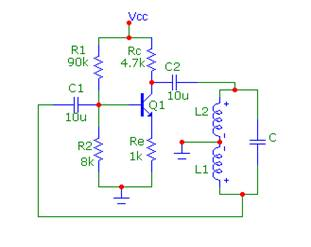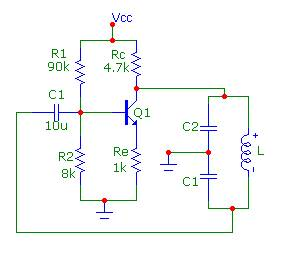EXPT NO:- 6

Title:- Simulate LC oscillators.

Aim:- To simulate LC (Hartley and colpitts) oscillators and compare the theoretical and practical

values of frequency of oscillations.

Pre-requisites : Principle of oscillator.

Types of Oscillators.

Equipments:- Computer with simulation tool Microcap

Theory:-

Oscillators -

An Oscillator basically consists of an amplifier and a phase shifting network. The amplifier receives the output of phase shifting network, amplifies it, phase shifts it through 1800 and applies it to the input of network. The network phase shifts the amplifier output through another 1800 and attenuates it before applying it back to amplifier input. Oscillators will not be sustained if, at the oscillator frequency, the magnitude of the product of the transfer gain of the amplifier and the magnitude of theFB factor of the FBN (the magnitude of the loop gain) are less than unity.

The condition of unity loop gain - Ab = 1 is called the Barkhausen criterion. Here Ã¯AbÃ¯= 1 and that phase of - Ab is zero.

LC Oscillators -

These oscillators use transistors and LC tuned circuits in their feedback network.A parallel resonant circuit is used to establish the oscillator frequency.There are two types of LC oscillators, Hartley and colpitts oscillator. In Hartley oscillators feedback is provided by a voltage divider between inductors L1 and L2 while in colpitts oscillator feedback is provided by a voltage divider between capacitors C1 and C2 .

Advantages of Phase Shift Oscillator:

1. Simplicity of the circuit.

2. Useful for frequencies in the audio range.

3. A sine wave output can be obtained.

Disadvantages of Phase Shift Oscillator:

1 Poor frequency stability : Frequency keeps changing as it depends on the values of R and C which can change due to factors such as temperature, humidity, aging etc.

2. It is difficult to get a variable frequency output, because to change the frequency, we need to vary all the resistors and capacitors simultaneously which is practically very difficult.

Circuit Diagram :- ( Hartley oscillator)Fig. a) Hartley Oscillator

Design: ( Hartley oscillator)

Vs=â€¦â€¦.. mV

Vo=â€¦â€¦..V

Av= Vo/Vs

AÎ² >=1

Î²=L1/L2

Let L1=1mH

C=10nF

L2=â€¦â€¦mH

Observation:

Hartley Oscillator : Time period (To)= â€¦â€¦â€¦â€¦uS

Calculations :

Circuit Diagram :- (Colpitts Oscillator)Fig. b) Colpitts Oscillator

Design: (Colpitts oscillator)

Vs=â€¦â€¦.. mV

Vo=â€¦â€¦..V

Av= Vo/Vs

AÎ² >=1

Î²=C2/C1

Let L=10mH

C2=10nF

C1=â€¦â€¦nF

Observation :

Colpitts Oscillator: Time period (To)= â€¦â€¦â€¦â€¦uS

RESULT TABLE :

 Sr.No. Name of the oscillator Frequency of oscillation(TH) Frequency of oscillation(PR) 01 02

COMMENTS AND CONCLUSION: ----------------------------------------------------------------------------------------------------------------------------------------------------------------------------------------------------------------------------------------------------------------------------------------------------------------------------------------------------------------------------------------------------------------------------------------------------------------------------------------------------------------------------------------------------------------------------------------------------------------------

FaceBook
Likes
Additonal Information